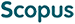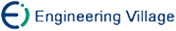迟利华 刘杰 胡庆丰. 数值并行计算可扩展性评价与测试[J]. 计算机研究与发展, 2005, 42(6): 1073-1078.
 引用本文: 迟利华 刘杰 胡庆丰. 数值并行计算可扩展性评价与测试[J]. 计算机研究与发展, 2005, 42(6): 1073-1078.Chi Lihua, Liu jie, and Hu Qingfeng. Evaluation and Test for Scalability of Numerical Parallel Computation[J]. Journal of Computer Research and Development, 2005, 42(6): 1073-1078.
 Citation: Chi Lihua, Liu jie, and Hu Qingfeng. Evaluation and Test for Scalability of Numerical Parallel Computation[J]. Journal of Computer Research and Development, 2005, 42(6): 1073-1078.## Evaluation and Test for Scalability of Numerical Parallel Computation

• 摘要: 分析了几种可扩展性能评价模型存在的问题，针对实际评价与测试的需要，提出了一种基于等平均负载的数值并行计算可扩展性评价模型.该评价模型对可扩展性能加速比和可扩展性进行了重新定义，给出了使用该模型的进行可扩展加速比和可扩展性测试的方法，结合曲线拟合或并行计算时间模型可以预测并行系统的可扩展性，对NPB BT，SP和矩阵乘法进行了可扩展性预测.

Abstract: In this paper, the existing problems of the current scalability models are analyzed, and aiming at the requirements of the practical evaluations and tests, a practical scalability metric based on iso-average-computation-load is proposed to provide a quantitative measurement of the scalability. In this metric, the definitions of scalable speedup and scalability are different from the current metrics. By using this metric, a practical method can be obtained to test scalable speedup and scalability, and combined with curve fitting or parallel computing time model, the scalabilities of parallel systems can be predicted./下载:  全尺寸图片 幻灯片
• 分享
• 用微信扫码二维码

分享至好友和朋友圈